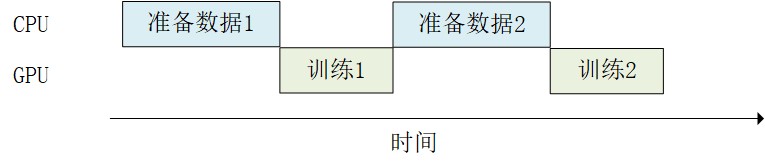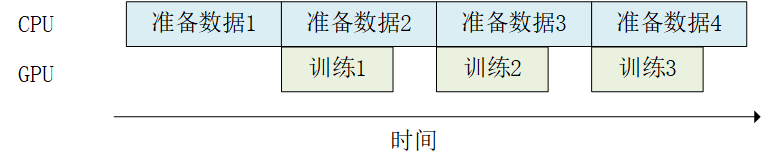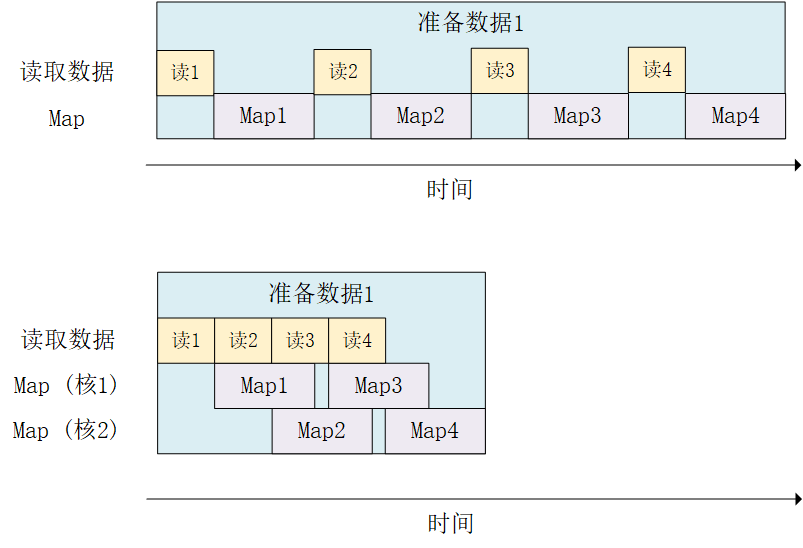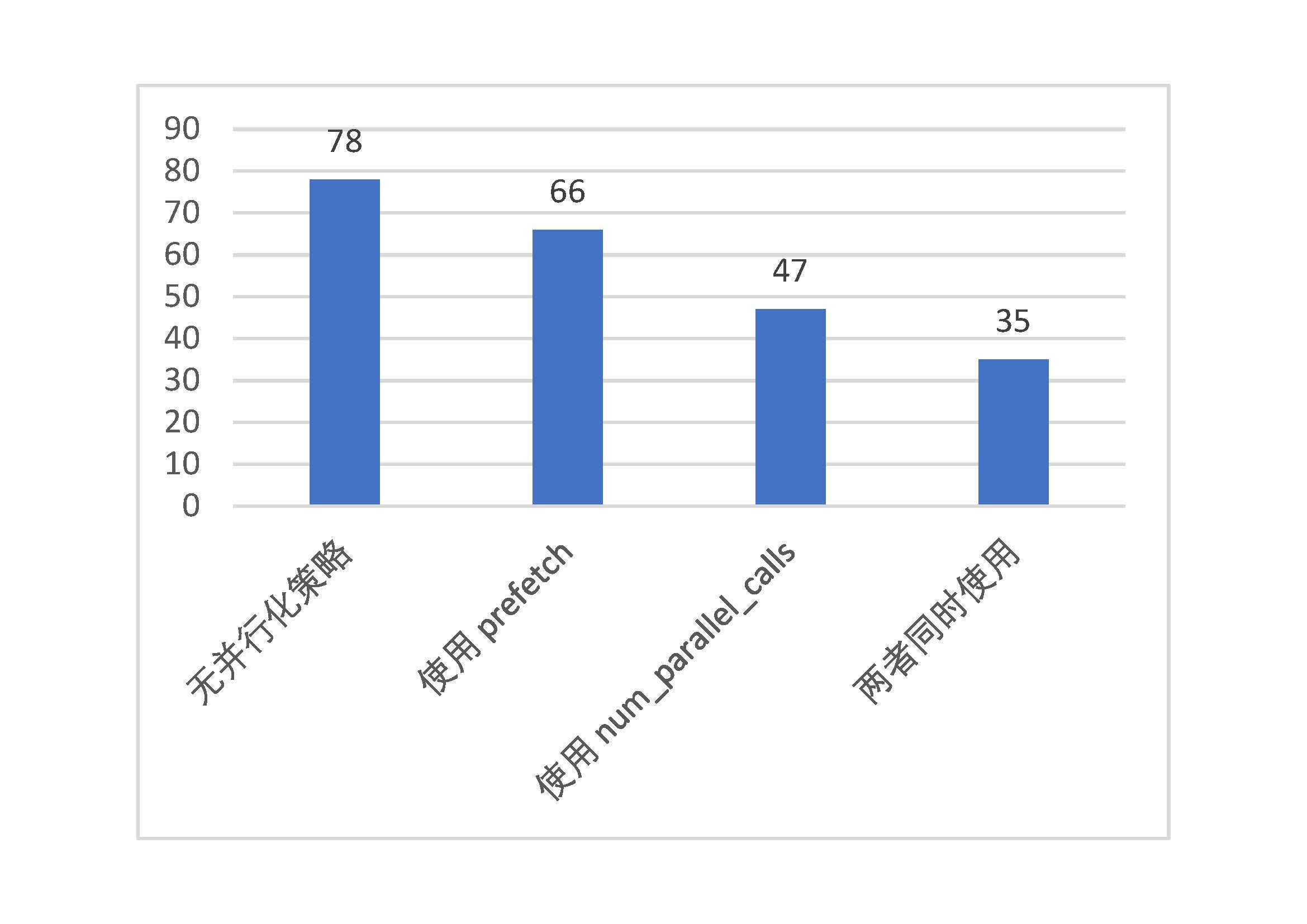# TensorFlow常用模块¶

## `tf.train.Checkpoint` ：变量的保存与恢复¶

Checkpoint只保存模型的参数，不保存模型的计算过程，因此一般用于在具有模型源代码的时候恢复之前训练好的模型参数。如果需要导出模型（无需源代码也能运行模型），请参考 “部署”章节中的SavedModel

```checkpoint = tf.train.Checkpoint(model=model)
```

```checkpoint = tf.train.Checkpoint(myAwesomeModel=model, myAwesomeOptimizer=optimizer)
```

```checkpoint.save(save_path_with_prefix)
```

```model_to_be_restored = MyModel()                                        # 待恢复参数的同一模型
checkpoint = tf.train.Checkpoint(myAwesomeModel=model_to_be_restored)   # 键名保持为“myAwesomeModel”
checkpoint.restore(save_path_with_prefix_and_index)
```

```# train.py 模型训练阶段

model = MyModel()
# 实例化Checkpoint，指定保存对象为model（如果需要保存Optimizer的参数也可加入）
checkpoint = tf.train.Checkpoint(myModel=model)
# ...（模型训练代码）
# 模型训练完毕后将参数保存到文件（也可以在模型训练过程中每隔一段时间就保存一次）
checkpoint.save('./save/model.ckpt')
```
```# test.py 模型使用阶段

model = MyModel()
checkpoint = tf.train.Checkpoint(myModel=model)             # 实例化Checkpoint，指定恢复对象为model
checkpoint.restore(tf.train.latest_checkpoint('./save'))    # 从文件恢复模型参数
# 模型使用代码
```

`tf.train.Checkpoint` 与以前版本常用的 `tf.train.Saver` 相比，强大之处在于其支持在即时执行模式下“延迟”恢复变量。具体而言，当调用了 `checkpoint.restore()` ，但模型中的变量还没有被建立的时候，Checkpoint可以等到变量被建立的时候再进行数值的恢复。即时执行模式下，模型中各个层的初始化和变量的建立是在模型第一次被调用的时候才进行的（好处在于可以根据输入的张量形状而自动确定变量形状，无需手动指定）。这意味着当模型刚刚被实例化的时候，其实里面还一个变量都没有，这时候使用以往的方式去恢复变量数值是一定会报错的。比如，你可以试试在train.py调用 `tf.keras.Model``save_weight()` 方法保存model的参数，并在test.py中实例化model后立即调用 `load_weight()` 方法，就会出错，只有当调用了一遍model之后再运行 `load_weight()` 方法才能得到正确的结果。可见， `tf.train.Checkpoint` 在这种情况下可以给我们带来相当大的便利。另外， `tf.train.Checkpoint` 同时也支持图执行模式。

```import tensorflow as tf
import numpy as np
import argparse
from zh.model.mnist.mlp import MLP

parser = argparse.ArgumentParser(description='Process some integers.')
args = parser.parse_args()

def train():
model = MLP()
num_batches = int(data_loader.num_train_data // args.batch_size * args.num_epochs)
checkpoint = tf.train.Checkpoint(myAwesomeModel=model)      # 实例化Checkpoint，设置保存对象为model
for batch_index in range(1, num_batches+1):
y_pred = model(X)
loss = tf.keras.losses.sparse_categorical_crossentropy(y_true=y, y_pred=y_pred)
loss = tf.reduce_mean(loss)
print("batch %d: loss %f" % (batch_index, loss.numpy()))
if batch_index % 100 == 0:                              # 每隔100个Batch保存一次
path = checkpoint.save('./save/model.ckpt')         # 保存模型参数到文件
print("model saved to %s" % path)

def test():
model_to_be_restored = MLP()
# 实例化Checkpoint，设置恢复对象为新建立的模型model_to_be_restored
checkpoint = tf.train.Checkpoint(myAwesomeModel=model_to_be_restored)
checkpoint.restore(tf.train.latest_checkpoint('./save'))    # 从文件恢复模型参数

if __name__ == '__main__':
if args.mode == 'train':
train()
if args.mode == 'test':
test()
```

• 在长时间的训练后，程序会保存大量的Checkpoint，但我们只想保留最后的几个Checkpoint；

• Checkpoint默认从1开始编号，每次累加1，但我们可能希望使用别的编号方式（例如使用当前Batch的编号作为文件编号）。

```checkpoint = tf.train.Checkpoint(model=model)
manager = tf.train.CheckpointManager(checkpoint, directory='./save', checkpoint_name='model.ckpt', max_to_keep=k)
```

```import tensorflow as tf
import numpy as np
import argparse
from zh.model.mnist.mlp import MLP

parser = argparse.ArgumentParser(description='Process some integers.')
args = parser.parse_args()

def train():
model = MLP()
num_batches = int(data_loader.num_train_data // args.batch_size * args.num_epochs)
checkpoint = tf.train.Checkpoint(myAwesomeModel=model)
# 使用tf.train.CheckpointManager管理Checkpoint
manager = tf.train.CheckpointManager(checkpoint, directory='./save', max_to_keep=3)
for batch_index in range(1, num_batches):
y_pred = model(X)
loss = tf.keras.losses.sparse_categorical_crossentropy(y_true=y, y_pred=y_pred)
loss = tf.reduce_mean(loss)
print("batch %d: loss %f" % (batch_index, loss.numpy()))
if batch_index % 100 == 0:
# 使用CheckpointManager保存模型参数到文件并自定义编号
path = manager.save(checkpoint_number=batch_index)
print("model saved to %s" % path)

def test():
model_to_be_restored = MLP()
checkpoint = tf.train.Checkpoint(myAwesomeModel=model_to_be_restored)
checkpoint.restore(tf.train.latest_checkpoint('./save'))

if __name__ == '__main__':
if args.mode == 'train':
train()
if args.mode == 'test':
test()
```

## TensorBoard：训练过程可视化¶

### 实时查看参数变化情况¶

```summary_writer = tf.summary.create_file_writer('./tensorboard')     # 参数为记录文件所保存的目录
```

```summary_writer = tf.summary.create_file_writer('./tensorboard')
# 开始模型训练
for batch_index in range(num_batches):
# ...（训练代码，当前batch的损失值放入变量loss中）
with summary_writer.as_default():                               # 希望使用的记录器
tf.summary.scalar("loss", loss, step=batch_index)
tf.summary.scalar("MyScalar", my_scalar, step=batch_index)  # 还可以添加其他自定义的变量
```

```tensorboard --logdir=./tensorboard
```

TensorBoard的使用有以下注意事项：

• 如果需要重新训练，需要删除掉记录文件夹内的信息并重启TensorBoard（或者建立一个新的记录文件夹并开启TensorBoard， `--logdir` 参数设置为新建立的文件夹）；

• 记录文件夹目录保持全英文。

### 查看Graph和Profile信息¶

```tf.summary.trace_on(graph=True, profiler=True)  # 开启Trace，可以记录图结构和profile信息
# 进行训练
with summary_writer.as_default():
tf.summary.trace_export(name="model_trace", step=0, profiler_outdir=log_dir)    # 保存Trace信息到文件
```

```pip install -U tensorboard-plugin-profile
```

### 实例：查看多层感知机模型的训练情况¶

```import tensorflow as tf
from zh.model.mnist.mlp import MLP

num_batches = 1000
batch_size = 50
learning_rate = 0.001
log_dir = 'tensorboard'
model = MLP()
summary_writer = tf.summary.create_file_writer(log_dir)     # 实例化记录器
tf.summary.trace_on(profiler=True)  # 开启Trace（可选）
for batch_index in range(num_batches):
y_pred = model(X)
loss = tf.keras.losses.sparse_categorical_crossentropy(y_true=y, y_pred=y_pred)
loss = tf.reduce_mean(loss)
print("batch %d: loss %f" % (batch_index, loss.numpy()))
with summary_writer.as_default():                           # 指定记录器
tf.summary.scalar("loss", loss, step=batch_index)       # 将当前损失函数的值写入记录器
with summary_writer.as_default():
tf.summary.trace_export(name="model_trace", step=0, profiler_outdir=log_dir)    # 保存Trace信息到文件（可选）
```

## `tf.data` ：数据集的构建与预处理¶

### 数据集对象的建立¶

`tf.data` 的核心是 `tf.data.Dataset` 类，提供了对数据集的高层封装。`tf.data.Dataset` 由一系列的可迭代访问的元素（element）组成，每个元素包含一个或多个张量。比如说，对于一个由图像组成的数据集，每个元素可以是一个形状为 `长×宽×通道数` 的图片张量，也可以是由图片张量和图片标签张量组成的元组（Tuple）。

```import tensorflow as tf
import numpy as np

X = tf.constant([2013, 2014, 2015, 2016, 2017])
Y = tf.constant([12000, 14000, 15000, 16500, 17500])

# 也可以使用NumPy数组，效果相同
# X = np.array([2013, 2014, 2015, 2016, 2017])
# Y = np.array([12000, 14000, 15000, 16500, 17500])

dataset = tf.data.Dataset.from_tensor_slices((X, Y))

for x, y in dataset:
print(x.numpy(), y.numpy())
```

```2013 12000
2014 14000
2015 15000
2016 16500
2017 17500
```

```import matplotlib.pyplot as plt

(train_data, train_label), (_, _) = tf.keras.datasets.mnist.load_data()
train_data = np.expand_dims(train_data.astype(np.float32) / 255.0, axis=-1)      # [60000, 28, 28, 1]
mnist_dataset = tf.data.Dataset.from_tensor_slices((train_data, train_label))

for image, label in mnist_dataset:
plt.title(label.numpy())
plt.imshow(image.numpy()[:, :, 0])
plt.show()
```

TensorFlow Datasets提供了一个基于 `tf.data.Datasets` 的开箱即用的数据集集合，相关内容可参考 TensorFlow Datasets 。例如，使用以下语句：

```import tensorflow_datasets as tfds
```

### 数据集对象的预处理¶

`tf.data.Dataset` 类为我们提供了多种数据集预处理方法。最常用的如：

• `Dataset.map(f)` ：对数据集中的每个元素应用函数 `f` ，得到一个新的数据集（这部分往往结合 `tf.io` 进行读写和解码文件， `tf.image` 进行图像处理）；

• `Dataset.shuffle(buffer_size)` ：将数据集打乱（设定一个固定大小的缓冲区（Buffer），取出前 `buffer_size` 个元素放入，并从缓冲区中随机采样，采样后的数据用后续数据替换）；

• `Dataset.batch(batch_size)` ：将数据集分成批次，即对每 `batch_size` 个元素，使用 `tf.stack()` 在第0维合并，成为一个元素；

```def rot90(image, label):
image = tf.image.rot90(image)
return image, label

mnist_dataset = mnist_dataset.map(rot90)

for image, label in mnist_dataset:
plt.title(label.numpy())
plt.imshow(image.numpy()[:, :, 0])
plt.show()

```

```mnist_dataset = mnist_dataset.batch(4)

for images, labels in mnist_dataset:    # image: [4, 28, 28, 1], labels: 
fig, axs = plt.subplots(1, 4)
for i in range(4):
axs[i].set_title(labels.numpy()[i])
axs[i].imshow(images.numpy()[i, :, :, 0])
plt.show()
```

```mnist_dataset = mnist_dataset.shuffle(buffer_size=10000).batch(4)

for images, labels in mnist_dataset:
fig, axs = plt.subplots(1, 4)
for i in range(4):
axs[i].set_title(labels.numpy()[i])
axs[i].imshow(images.numpy()[i, :, :, 0])
plt.show()
```

`Dataset.shuffle()` 时缓冲区大小 `buffer_size` 的设置

`tf.data.Dataset` 作为一个针对大规模数据设计的迭代器，本身无法方便地获得自身元素的数量或随机访问元素。因此，为了高效且较为充分地打散数据集，需要一些特定的方法。`Dataset.shuffle()` 采取了以下方法：

• 设定一个固定大小为 `buffer_size` 的缓冲区（Buffer）；

• 初始化时，取出数据集中的前 `buffer_size` 个元素放入缓冲区；

• 每次需要从数据集中取元素时，即从缓冲区中随机采样一个元素并取出，然后从后续的元素中取出一个放回到之前被取出的位置，以维持缓冲区的大小。

• `buffer_size` 设置为1时，其实等价于没有进行任何打散；

• 当数据集的标签顺序分布极为不均匀（例如二元分类时数据集前N个的标签为0，后N个的标签为1）时，较小的缓冲区大小会使得训练时取出的Batch数据很可能全为同一标签，从而影响训练效果。一般而言，数据集的顺序分布若较为随机，则缓冲区的大小可较小，否则则需要设置较大的缓冲区。

### 使用 `tf.data` 的并行化策略提高训练流程效率¶`Dataset.prefetch()` 的使用方法和前节的 `Dataset.batch()``Dataset.shuffle()` 等非常类似。继续以前节的MNIST数据集为例，若希望开启预加载数据，使用如下代码即可：

```mnist_dataset = mnist_dataset.prefetch(buffer_size=tf.data.experimental.AUTOTUNE)
```

```mnist_dataset = mnist_dataset.map(map_func=rot90, num_parallel_calls=2)
```### 数据集元素的获取与使用¶

```dataset = tf.data.Dataset.from_tensor_slices((A, B, C, ...))
for a, b, c, ... in dataset:
# 对张量a, b, c等进行操作，例如送入模型进行训练
```

```dataset = tf.data.Dataset.from_tensor_slices((A, B, C, ...))
it = iter(dataset)
a_0, b_0, c_0, ... = next(it)
a_1, b_1, c_1, ... = next(it)
```

Keras支持使用 `tf.data.Dataset` 直接作为输入。当调用 `tf.keras.Model``fit()``evaluate()` 方法时，可以将参数中的输入数据 `x` 指定为一个元素格式为 `(输入数据, 标签数据)``Dataset` ，并忽略掉参数中的标签数据 `y` 。例如，对于上述的MNIST数据集，常规的Keras训练方式是：

```model.fit(x=train_data, y=train_label, epochs=num_epochs, batch_size=batch_size)
```

```model.fit(mnist_dataset, epochs=num_epochs)
```

### 实例：cats_vs_dogs图像分类¶

```import tensorflow as tf
import os

num_epochs = 10
batch_size = 32
learning_rate = 0.001
data_dir = 'C:/datasets/cats_vs_dogs'
train_cats_dir = data_dir + '/train/cats/'
train_dogs_dir = data_dir + '/train/dogs/'
test_cats_dir = data_dir + '/valid/cats/'
test_dogs_dir = data_dir + '/valid/dogs/'

def _decode_and_resize(filename, label):
image_decoded = tf.image.decode_jpeg(image_string)  # 解码JPEG图片
image_resized = tf.image.resize(image_decoded, [256, 256]) / 255.0
return image_resized, label

if __name__ == '__main__':
# 构建训练数据集
train_cat_filenames = tf.constant([train_cats_dir + filename for filename in os.listdir(train_cats_dir)])
train_dog_filenames = tf.constant([train_dogs_dir + filename for filename in os.listdir(train_dogs_dir)])
train_filenames = tf.concat([train_cat_filenames, train_dog_filenames], axis=-1)
train_labels = tf.concat([
tf.zeros(train_cat_filenames.shape, dtype=tf.int32),
tf.ones(train_dog_filenames.shape, dtype=tf.int32)],
axis=-1)

train_dataset = tf.data.Dataset.from_tensor_slices((train_filenames, train_labels))
train_dataset = train_dataset.map(
map_func=_decode_and_resize,
num_parallel_calls=tf.data.experimental.AUTOTUNE)
# 取出前buffer_size个数据放入buffer，并从其中随机采样，采样后的数据用后续数据替换
train_dataset = train_dataset.shuffle(buffer_size=23000)
train_dataset = train_dataset.batch(batch_size)
train_dataset = train_dataset.prefetch(tf.data.experimental.AUTOTUNE)

model = tf.keras.Sequential([
tf.keras.layers.Conv2D(32, 3, activation='relu', input_shape=(256, 256, 3)),
tf.keras.layers.MaxPooling2D(),
tf.keras.layers.Conv2D(32, 5, activation='relu'),
tf.keras.layers.MaxPooling2D(),
tf.keras.layers.Flatten(),
tf.keras.layers.Dense(64, activation='relu'),
tf.keras.layers.Dense(2, activation='softmax')
])

model.compile(
loss=tf.keras.losses.sparse_categorical_crossentropy,
metrics=[tf.keras.metrics.sparse_categorical_accuracy]
)

model.fit(train_dataset, epochs=num_epochs)
```

```    # 构建测试数据集
test_cat_filenames = tf.constant([test_cats_dir + filename for filename in os.listdir(test_cats_dir)])
test_dog_filenames = tf.constant([test_dogs_dir + filename for filename in os.listdir(test_dogs_dir)])
test_filenames = tf.concat([test_cat_filenames, test_dog_filenames], axis=-1)
test_labels = tf.concat([
tf.zeros(test_cat_filenames.shape, dtype=tf.int32),
tf.ones(test_dog_filenames.shape, dtype=tf.int32)],
axis=-1)

test_dataset = tf.data.Dataset.from_tensor_slices((test_filenames, test_labels))
test_dataset = test_dataset.map(_decode_and_resize)
test_dataset = test_dataset.batch(batch_size)

print(model.metrics_names)
print(model.evaluate(test_dataset))
```tf.data 的并行化策略性能测试（纵轴为每epoch训练所需时间，单位：秒）

## TFRecord ：TensorFlow数据集存储格式¶

TFRecord 是TensorFlow 中的数据集存储格式。当我们将数据集整理成 TFRecord 格式后，TensorFlow就可以高效地读取和处理这些数据集，从而帮助我们更高效地进行大规模的模型训练。

TFRecord可以理解为一系列序列化的 `tf.train.Example` 元素所组成的列表文件，而每一个 `tf.train.Example` 又由若干个 `tf.train.Feature` 的字典组成。形式如下：

```# dataset.tfrecords
[
{   # example 1 (tf.train.Example)
'feature_1': tf.train.Feature,
...
'feature_k': tf.train.Feature
},
...
{   # example N (tf.train.Example)
'feature_1': tf.train.Feature,
...
'feature_k': tf.train.Feature
}
]
```

• 读取该数据元素到内存；

• 将该元素转换为 `tf.train.Example` 对象（每一个 `tf.train.Example` 由若干个 `tf.train.Feature` 的字典组成，因此需要先建立Feature的字典）；

• 将该 `tf.train.Example` 对象序列化为字符串，并通过一个预先定义的 `tf.io.TFRecordWriter` 写入 TFRecord 文件。

• 通过 `tf.data.TFRecordDataset` 读入原始的 TFRecord 文件（此时文件中的 `tf.train.Example` 对象尚未被反序列化），获得一个 `tf.data.Dataset` 数据集对象；

• 通过 `Dataset.map` 方法，对该数据集对象中的每一个序列化的 `tf.train.Example` 字符串执行 `tf.io.parse_single_example` 函数，从而实现反序列化。

### 将数据集存储为 TFRecord 文件¶

```import tensorflow as tf
import os

data_dir = 'C:/datasets/cats_vs_dogs'
train_cats_dir = data_dir + '/train/cats/'
train_dogs_dir = data_dir + '/train/dogs/'
tfrecord_file = data_dir + '/train/train.tfrecords'

train_cat_filenames = [train_cats_dir + filename for filename in os.listdir(train_cats_dir)]
train_dog_filenames = [train_dogs_dir + filename for filename in os.listdir(train_dogs_dir)]
train_filenames = train_cat_filenames + train_dog_filenames
train_labels =  * len(train_cat_filenames) +  * len(train_dog_filenames)  # 将 cat 类的标签设为0，dog 类的标签设为1
```

```with tf.io.TFRecordWriter(tfrecord_file) as writer:
for filename, label in zip(train_filenames, train_labels):
image = open(filename, 'rb').read()     # 读取数据集图片到内存，image 为一个 Byte 类型的字符串
feature = {                             # 建立 tf.train.Feature 字典
'image': tf.train.Feature(bytes_list=tf.train.BytesList(value=[image])),  # 图片是一个 Bytes 对象
'label': tf.train.Feature(int64_list=tf.train.Int64List(value=[label]))   # 标签是一个 Int 对象
}
example = tf.train.Example(features=tf.train.Features(feature=feature)) # 通过字典建立 Example
writer.write(example.SerializeToString())   # 将Example序列化并写入 TFRecord 文件
```

• `tf.train.BytesList` ：字符串或原始Byte文件（如图片），通过 `bytes_list` 参数传入一个由字符串数组初始化的 `tf.train.BytesList` 对象；

• `tf.train.FloatList` ：浮点数，通过 `float_list` 参数传入一个由浮点数数组初始化的 `tf.train.FloatList` 对象；

• `tf.train.Int64List` ：整数，通过 `int64_list` 参数传入一个由整数数组初始化的 `tf.train.Int64List` 对象。

### 读取 TFRecord 文件¶

```raw_dataset = tf.data.TFRecordDataset(tfrecord_file)    # 读取 TFRecord 文件

feature_description = { # 定义Feature结构，告诉解码器每个Feature的类型是什么
'image': tf.io.FixedLenFeature([], tf.string),
'label': tf.io.FixedLenFeature([], tf.int64),
}

def _parse_example(example_string): # 将 TFRecord 文件中的每一个序列化的 tf.train.Example 解码
feature_dict = tf.io.parse_single_example(example_string, feature_description)
feature_dict['image'] = tf.io.decode_jpeg(feature_dict['image'])    # 解码JPEG图片
return feature_dict['image'], feature_dict['label']

dataset = raw_dataset.map(_parse_example)
```

```import matplotlib.pyplot as plt

for image, label in dataset:
plt.title('cat' if label == 0 else 'dog')
plt.imshow(image.numpy())
plt.show()
```

## `tf.function` ：图执行模式 *¶

### `tf.function` 基础使用方法¶

```import tensorflow as tf
import time
from zh.model.mnist.cnn import CNN

num_batches = 1000
batch_size = 50
learning_rate = 0.001
model = CNN()

@tf.function
def train_one_step(X, y):
y_pred = model(X)
loss = tf.keras.losses.sparse_categorical_crossentropy(y_true=y, y_pred=y_pred)
loss = tf.reduce_mean(loss)
# 注意这里使用了TensorFlow内置的tf.print()。@tf.function不支持Python内置的print方法
tf.print("loss", loss)

start_time = time.time()
for batch_index in range(num_batches):
train_one_step(X, y)
end_time = time.time()
print(end_time - start_time)
```

### `tf.function` 内在机制¶

• 在即时执行模式关闭的环境下，函数内的代码依次运行。也就是说，每个 `tf.` 方法都只是定义了计算节点，而并没有进行任何实质的计算。这与TensorFlow 1.X的图执行模式是一致的；

• 使用AutoGraph将函数中的Python控制流语句转换成TensorFlow计算图中的对应节点（比如说 `while``for` 语句转换为 `tf.while``if` 语句转换为 `tf.cond` 等等；

• 基于上面的两步，建立函数内代码的计算图表示（为了保证图的计算顺序，图中还会自动加入一些 `tf.control_dependencies` 节点）；

• 运行一次这个计算图；

• 基于函数的名字和输入的函数参数的类型生成一个哈希值，并将建立的计算图缓存到一个哈希表中。

```graph = train_one_step.get_concrete_function(X, y)
```

```import tensorflow as tf
import numpy as np

@tf.function
def f(x):
print("The function is running in Python")
tf.print(x)

a = tf.constant(1, dtype=tf.int32)
f(a)
b = tf.constant(2, dtype=tf.int32)
f(b)
b_ = np.array(2, dtype=np.int32)
f(b_)
c = tf.constant(0.1, dtype=tf.float32)
f(c)
d = tf.constant(0.2, dtype=tf.float32)
f(d)
```

```The function is running in Python
1
2
2
The function is running in Python
0.1
0.2
```

• 将函数内的代码依次运行了一遍（因此输出了文本）；

• 构建了计算图，然后运行了一次该计算图（因此输出了1）。这里 `tf.print(x)` 可以作为计算图的节点，但Python内置的 `print` 则不能被转换成计算图的节点。因此，计算图中只包含了 `tf.print(x)` 这一操作；

• 将该计算图缓存到了一个哈希表中（如果之后再有类型为 `tf.int32` ，shape为空的张量输入，则重复使用已构建的计算图）。

```f(d)
f(1)
f(2)
f(1)
f(0.1)
f(0.2)
f(0.1)
```

```The function is running in Python
1
The function is running in Python
2
1
The function is running in Python
0.1
The function is running in Python
0.2
0.1
```

```import tensorflow as tf

a = tf.Variable(0.0)

@tf.function
def g():
a.assign(a + 1.0)
return a

print(g())
print(g())
print(g())
```

```tf.Tensor(1.0, shape=(), dtype=float32)
tf.Tensor(2.0, shape=(), dtype=float32)
tf.Tensor(3.0, shape=(), dtype=float32)
```

### AutoGraph：将Python控制流转换为TensorFlow计算图¶

```import tensorflow as tf

@tf.function
def square_if_positive(x):
if x > 0:
x = x * x
else:
x = 0
return x

a = tf.constant(1)
b = tf.constant(-1)
print(square_if_positive(a), square_if_positive(b))
print(tf.autograph.to_code(square_if_positive.python_function))
```

```tf.Tensor(1, shape=(), dtype=int32) tf.Tensor(0, shape=(), dtype=int32)
def tf__square_if_positive(x):
do_return = False
retval_ = ag__.UndefinedReturnValue()
cond = x > 0

def get_state():
return ()

def set_state(_):
pass

def if_true():
x_1, = x,
x_1 = x_1 * x_1
return x_1

def if_false():
x = 0
return x
x = ag__.if_stmt(cond, if_true, if_false, get_state, set_state)
do_return = True
retval_ = x
cond_1 = ag__.is_undefined_return(retval_)

def get_state_1():
return ()

def set_state_1(_):
pass

def if_true_1():
retval_ = None
return retval_

def if_false_1():
return retval_
retval_ = ag__.if_stmt(cond_1, if_true_1, if_false_1, get_state_1, set_state_1)
return retval_
```

### 使用传统的 `tf.Session`¶

```    optimizer = tf.compat.v1.train.AdamOptimizer(learning_rate=learning_rate)
num_batches = int(data_loader.num_train_data // batch_size * num_epochs)
# 建立计算图
X_placeholder = tf.compat.v1.placeholder(name='X', shape=[None, 28, 28, 1], dtype=tf.float32)
y_placeholder = tf.compat.v1.placeholder(name='y', shape=[None], dtype=tf.int32)
y_pred = model(X_placeholder)
loss = tf.keras.losses.sparse_categorical_crossentropy(y_true=y_placeholder, y_pred=y_pred)
loss = tf.reduce_mean(loss)
train_op = optimizer.minimize(loss)
sparse_categorical_accuracy = tf.keras.metrics.SparseCategoricalAccuracy()
# 建立Session
with tf.compat.v1.Session() as sess:
sess.run(tf.compat.v1.global_variables_initializer())
for batch_index in range(num_batches):
# 使用Session.run()将数据送入计算图节点，进行训练以及计算损失函数
_, loss_value = sess.run([train_op, loss], feed_dict={X_placeholder: X, y_placeholder: y})
print("batch %d: loss %f" % (batch_index, loss_value))

for batch_index in range(num_batches):
start_index, end_index = batch_index * batch_size, (batch_index + 1) * batch_size
print("test accuracy: %f" % sess.run(sparse_categorical_accuracy.result()))
```

## `tf.TensorArray` ：TensorFlow 动态数组 *¶

• `arr = tf.TensorArray(dtype, size, dynamic_size=False)` ：声明一个大小为 `size` ，类型为 `dtype` 的TensorArray `arr` 。如果将 `dynamic_size` 参数设置为 `True` ，则该数组会自动增长空间。

• `write(index, value)` ：将 `value` 写入数组的第 `index` 个位置；

• `read(index)` ：读取数组的第 `index` 个值；

```arr = arr.write(index, value)
```

```arr.write(index, value)     # 生成的计算图操作没有左值接收，从而丢失
```

```import tensorflow as tf

@tf.function
arr = tf.TensorArray(dtype=tf.float32, size=3)
arr = arr.write(0, tf.constant(0.0))
arr = arr.write(1, tf.constant(1.0))
arr = arr.write(2, tf.constant(2.0))
return arr_0, arr_1, arr_2

print(a, b, c)
```

```tf.Tensor(0.0, shape=(), dtype=float32) tf.Tensor(1.0, shape=(), dtype=float32) tf.Tensor(2.0, shape=(), dtype=float32)
```

## `tf.config`：GPU的使用与分配 *¶

### 指定当前程序使用的GPU¶

```gpus = tf.config.list_physical_devices(device_type='GPU')
cpus = tf.config.list_physical_devices(device_type='CPU')
print(gpus, cpus)
```

```[PhysicalDevice(name='/physical_device:GPU:0', device_type='GPU'),
PhysicalDevice(name='/physical_device:GPU:1', device_type='GPU'),
PhysicalDevice(name='/physical_device:GPU:2', device_type='GPU'),
PhysicalDevice(name='/physical_device:GPU:3', device_type='GPU')]
[PhysicalDevice(name='/physical_device:CPU:0', device_type='CPU')]
```

```gpus = tf.config.list_physical_devices(device_type='GPU')
tf.config.set_visible_devices(devices=gpus[0:2], device_type='GPU')
```

```tf.config.set_visible_devices(devices=[], device_type='GPU')
```

```export CUDA_VISIBLE_DEVICES=2,3
```

```import os
os.environ['CUDA_VISIBLE_DEVICES'] = "2,3"
```

### 设置显存使用策略¶

• 仅在需要时申请显存空间（程序初始运行时消耗很少的显存，随着程序的运行而动态申请显存）；

• 限制消耗固定大小的显存（程序不会超出限定的显存大小，若超出的报错）。

```gpus = tf.config.list_physical_devices(device_type='GPU')
for gpu in gpus:
tf.config.experimental.set_memory_growth(device=gpu, enable=True)
```

```gpus = tf.config.list_physical_devices(device_type='GPU')
tf.config.set_logical_device_configuration(
gpus,
[tf.config.LogicalDeviceConfiguration(memory_limit=1024)])
```

TensorFlow 1.X 的 图执行模式 下，可以在实例化新的session时传入 `tf.compat.v1.ConfigPhoto` 类来设置TensorFlow使用显存的策略。具体方式是实例化一个 `tf.ConfigProto` 类，设置参数，并在创建 `tf.compat.v1.Session` 时指定Config参数。以下代码通过 `allow_growth` 选项设置TensorFlow仅在需要时申请显存空间：

```config = tf.compat.v1.ConfigProto()
config.gpu_options.allow_growth = True
sess = tf.compat.v1.Session(config=config)
```

```config = tf.compat.v1.ConfigProto()
config.gpu_options.per_process_gpu_memory_fraction = 0.4
tf.compat.v1.Session(config=config)
```

### 单GPU模拟多GPU环境¶

```gpus = tf.config.list_physical_devices('GPU')
tf.config.set_logical_device_configuration(
gpus,
[tf.config.LogicalDeviceConfiguration(memory_limit=2048),
tf.config.LogicalDeviceConfiguration(memory_limit=2048)])
```

```Number of devices: 2
```